# KPI异常检测【一】- 时间序列分解算法

1、相关概念

2 、常见的时间序列

3、时间序列分解

3.1 方法介绍

3.2 经典方法

3.3 Holt Winter 指数平滑

3.4 STL分解

4 、异常准则

5、 异常检测算法

# 1、相关概念

## 1.1 异常

|预测值-真实值| > 阈值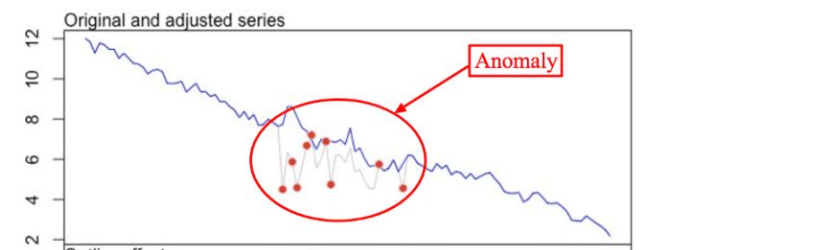## 1.2 时间序列

### 1.2.1 组成

(1)现象所属的时间；

(2)现象在不同时间上的观测值。现象在不同时间上的观测值的表现形式，有绝对数、相对数和平均数。

# 2、常见的时间序列

## 2.1 恒值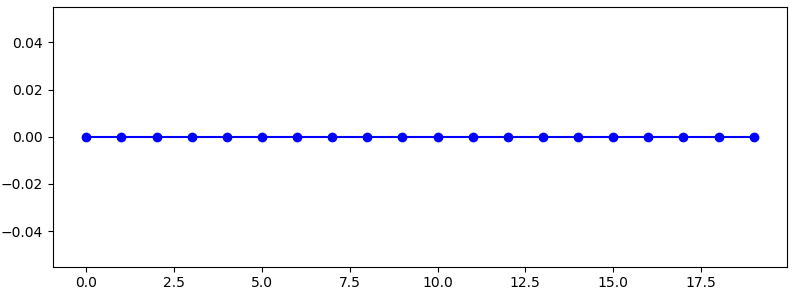y = np.zeros(20)
plt.figure(figsize=(8, 3))
plt.plot(y, color='b', marker='o')
plt.show()

## 2.2 趋势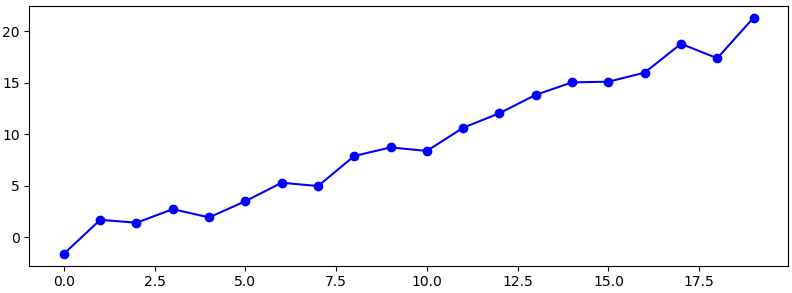n = 20
x = np.arange(n)
y_s = list(x)
u, sigma = 0.0, 1.0
e = np.random.normal(loc=u, scale=sigma, size=n)
y = y_s + e
plt.figure(figsize=(8, 3))
plt.plot(y, color='b', marker='o')
plt.show()


## 2.3 白噪声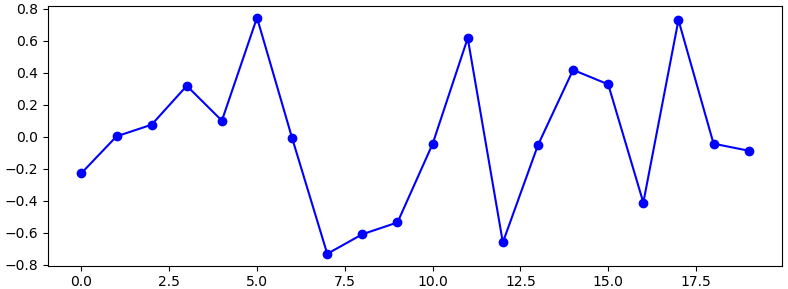n = 20
x = np.arange(n)
y = np.random.normal(0, 0.5, 20)
plt.figure(figsize=(8, 3))
plt.plot(y, color='b', marker='o')
plt.show()

## 2.4 周期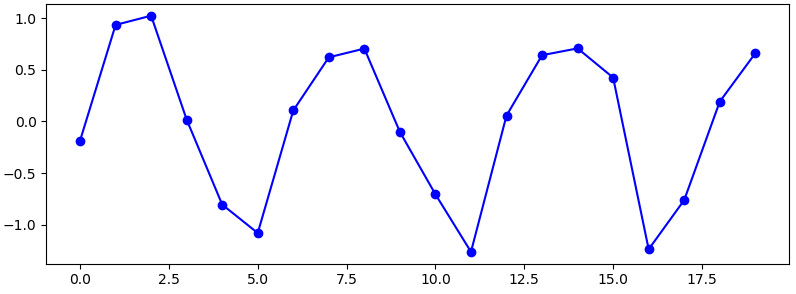n = 20
x = np.arange(n)
noise = np.random.normal(0, 0.2, 20)
season = np.array([math.sin(i/3*math.pi) for i in x])
y = season + noise
plt.figure(figsize=(8, 3))
plt.plot(y, color='b', marker='o')
plt.show()

## 2.5 周期+趋势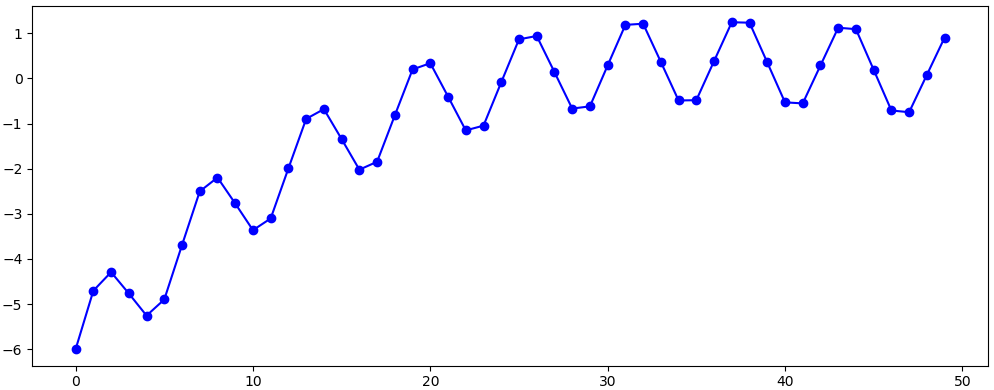np.random.seed(0)
x = np.arange(start=0, step=0.04, stop=2)
trend = [(a-1)*(a-2)*(a-3) for a in x]
noise = np.random.normal(0, 0.3, len(x))
season = np.array([math.sin(i/3*math.pi) for i in range(len(x))])
y = trend + season
plt.figure(figsize=(10, 4))
plt.plot(y, color='b', marker='o')
plt.show()

# 3、时间序列分解

## 3.1 方法介绍

### 3.1.1 目的

1. 寻找序列中的周期性和趋势性
2. 进行时序预测
3. 异常检测

### 3.1.3 模型

• 　    Y——需求预测
• 　　T——趋势
• 　　S——季节
• 　　R——残差

• 当周期性不随趋势而变化时，选加法模型，否则选乘法模型；
• 当目标有负值时，选加法模型；
• 当处理的是经济相关数据时，选乘法模型，增加可解释性。

### 3.1.4 方法

1. 经典方法
2. Holt-Winters方法
3. STL（Seasonal-Trend decomposition procedure based on Loess）方法

## 3.2 经典方法

### 3.2.1 算法步骤

1. 使用滑动平均，减去滑动平均的均值，得到趋势；
2. 计算趋势序列
3. 在趋势序列中，间隔为周期的序列求均值，得到周期；
4. 标准化周期数据，即和为0；
5. 求残差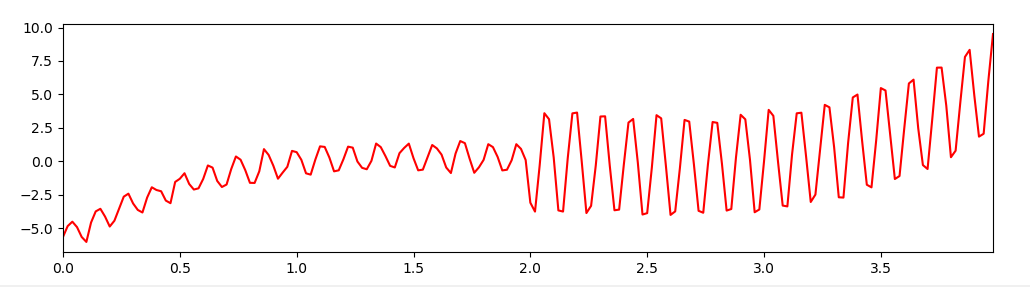# 数据准备-变周期
np.random.seed(0)
x = np.arange(start=0, step=0.02, stop=4)
trend = [(a - 1) * (a - 2) * (a - 3) for a in x]
season = np.array([math.sin(i / 3 * math.pi) for i in range(len(x))])
noise = np.random.normal(0, 0.2, len(x))
y = trend + season + noise
plt.figure(figsize=(12, 5))
pd.Series(data=y, index=x).plot(color='r', linestyle='-')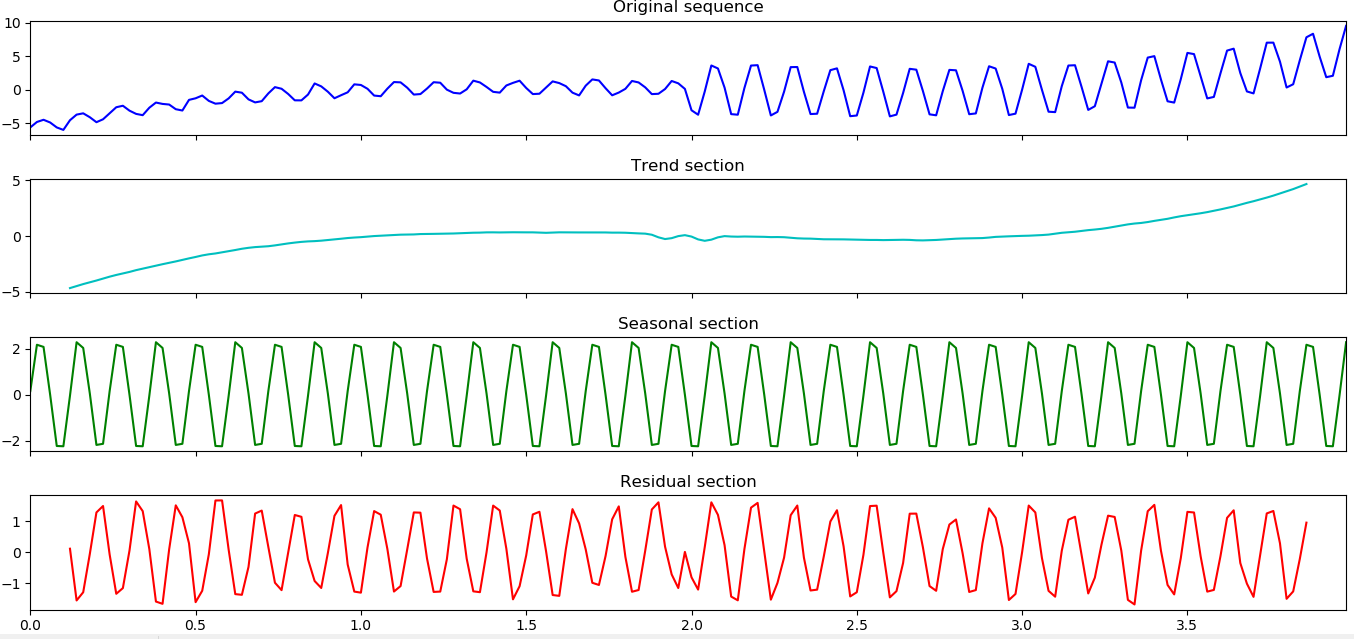# 模型展示
from statsmodels.tsa import seasonal
fig, ax_arr = plt.subplots(4, sharex=True)
fig.set_size_inches(12, 8)

pd.Series(data=y, index=x).plot(ax=ax_arr, color="b", linestyle='-')
ax_arr.set_title("Original sequence")

pd.Series(data=decompose_model.trend, index=x).plot(ax=ax_arr, color="c", linestyle='-')
ax_arr.set_title("Trend section")

pd.Series(data=decompose_model.seasonal, index=x).plot(ax=ax_arr, color="g", linestyle='-')
ax_arr.set_title("Seasonal section")

pd.Series(data=decompose_model.resid, index=x).plot(ax=ax_arr, color="r", linestyle='-')
ax_arr.set_title("Residual section")

plt.show()

### 3.2.2 优缺点

• 简单，算法研究的基础

• 前后时刻的值有缺失，无法预测
• 不能识别变周期
• 对异常点敏感

## 3.3 Holt Winter 指数平滑

• 一次指数平滑法——>针对没有趋势和季节性的序列
• 二次指数平滑法——>针对有趋势但是没有季节特性的时间序列
• 三次指数平滑法——>可以预测具有趋势和季节性的时间序列。术语“Holt-Winter”指的是三次指数平滑。

### 3.3.1  一次指数平滑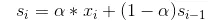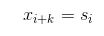### 3.2.2 二次指数平滑法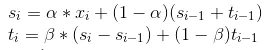t_i代表平滑后的趋势，当前趋势的未平滑值是当前平滑值s_i和上一个平滑值s_{i-1}的差。s_i为当前平滑值，是在一次指数平滑基础上加入了上一步的趋势信息t_{i-1}。利用这种方法做预测，就取最后的平滑值，然后每增加一个时间步长，就在该平滑值上增加一个t_{i}：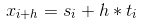### 3.2.3 Holt-Winter指数平滑法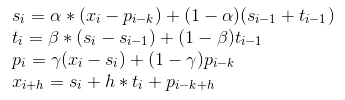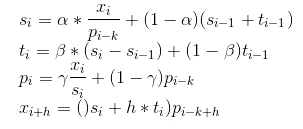### 3.2.4 优缺点

• 拟合趋势和周期
• 快速响应趋势和周期的变化

• 异常点影响大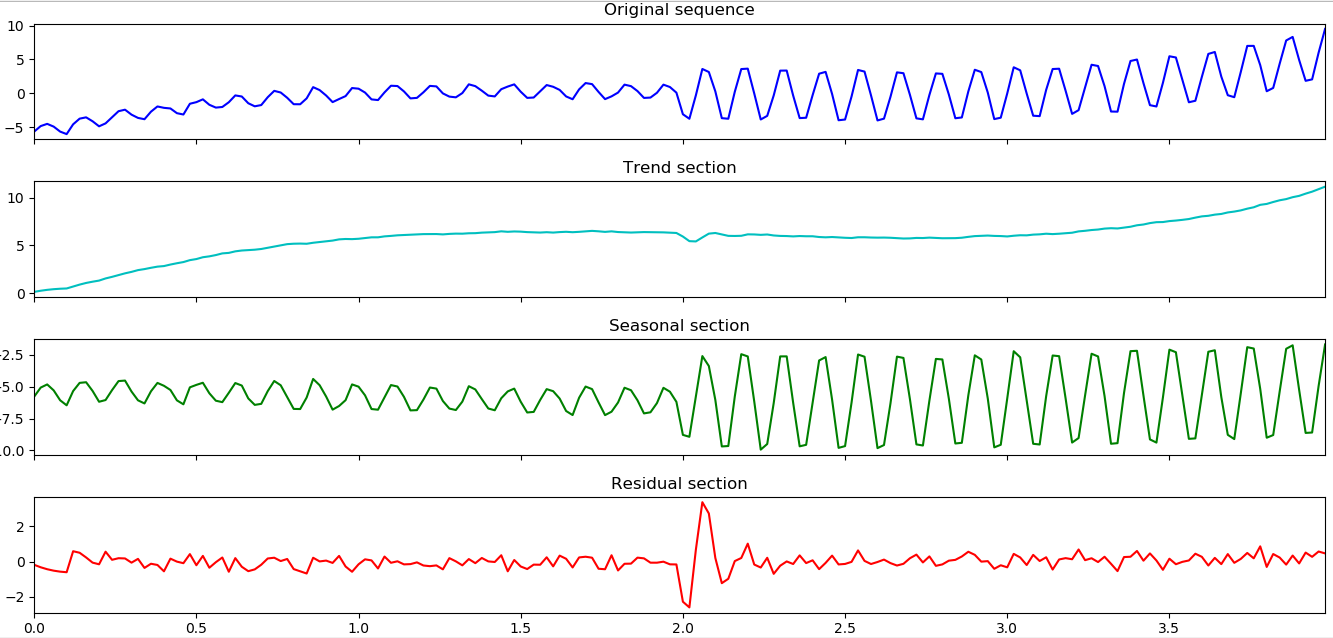# 数据同上
# 模型展示
from statsmodels.tsa.api import ExponentialSmoothing
fig, ax_arr = plt.subplots(4, sharex=True)
fig.set_size_inches(12, 8)

pd.Series(data=y, index=x).plot(ax=ax_arr, color="b", linestyle='-')
ax_arr.set_title("Original sequence")

pd.Series(data=holt_winter_model.level, index=x).plot(ax=ax_arr, color="c", linestyle='-')
ax_arr.set_title("Trend section")

pd.Series(data=holt_winter_model.season, index=x).plot(ax=ax_arr, color="g", linestyle='-')
ax_arr.set_title("Seasonal section")

pd.Series(data=holt_winter_model.resid, index=x).plot(ax=ax_arr, color="r", linestyle='-')
ax_arr.set_title("Residual section")

plt.show()

## 3.4 STL分解

STL(’Seasonal and Trend decomposition using Loess‘ )是以鲁棒局部加权回归作为平滑方法的时间序列分解方法。

其中Loess(locally weighted scatterplot smoothing,LOWESS or LOESS)为局部多项式回归拟合，是对两维散点图

STL的具体流程如下：

outer loop:
计算robustness weight;
inner loop:
Step 1 去趋势;
Step 2 周期子序列平滑;
Step 3 周期子序列的低通量过滤;
Step 4 去除平滑周期子序列趋势;
Step 5 去周期;
Step 6 趋势平滑;

Yv=Tv+Sv+Rv=1,⋯,N

STL分为内循环（inner loop）与外循环（outer loop），其中内循环主要做了趋势拟合与周期分量的计算。假定为内循环中第k-1次pass结束时的趋势分量、周期分量，初始时；并有以下参数：

• n(i)内层循环数，
• n(o)外层循环数，
• n(p)为一个周期的样本数，
• n(s)为Step 2中LOESS平滑参数，
• n(l)n为Step 3中LOESS平滑参数，
• n(t)为Step 6中LOESS平滑参数。

• Step 1: 去趋势（Detrending），减去上一轮结果的趋势分量，
• Step 2: 周期子序列平滑（Cycle-subseries smoothing），用LOESS ()对每个子序列做回归，并向前向后各延展一个周期；平滑结果组成temporary seasonal series，记为;
• Step 3: 周期子序列的低通量过滤（Low-Pass Filtering），对上一个步骤的结果序列依次做长度为2*n(p)、3的滑动平均（moving average），然后做LOESS ()回归，得到结果序列;
• Step 4: 去除平滑周期子序列趋势（Detrending of Smoothed Cycle-subseries）,得到周期
• Step 5: 去周期（Deseasonalizing），减去周期分量，
• Step 6: 趋势平滑（Trend Smoothing），对于去除周期之后的序列做LOESS ()回归，得到趋势分量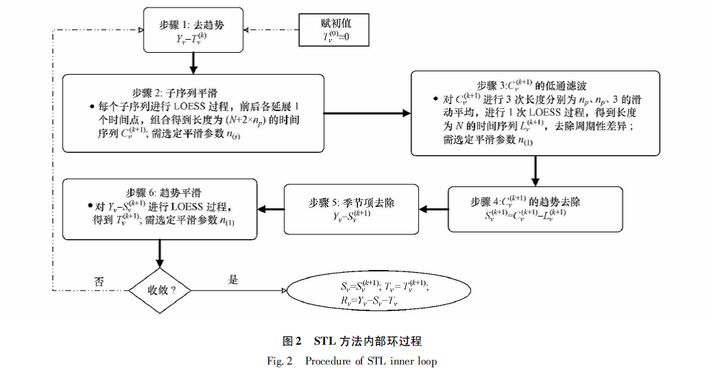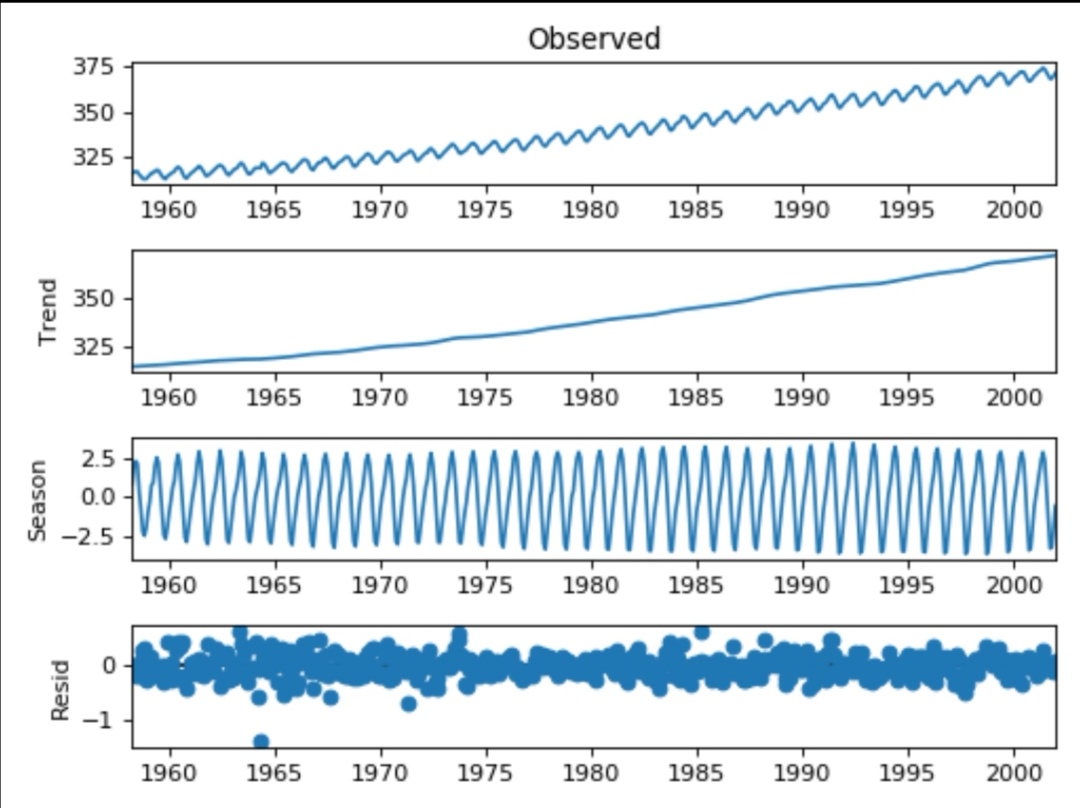# pip install git+https://github.com/statsmodels/statsmodels.git
from statsmodels.tsa.seasonal import STL
stl = STL(y, freq=6).fit()
fig = stl.plot()
fig.set_size_inches(12, 8)
fig.set_tight_layout(True)
plt.show()

# 4、异常准则

## 4.1 n-sigma准则

n-sigma准则基于目标分布是正态或近似正态分布。一般地，选取n=3。根据正态分布的概率密度公式可以算出，样本取值几乎全部(99.7%)集中在 区间内，其中，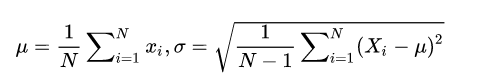1. 检测突增异常：异常区间为  ;
2. 检测突降异常：异常区间为  ;
3. 检测双向（突增或突降）异常：  ， 如下图所示;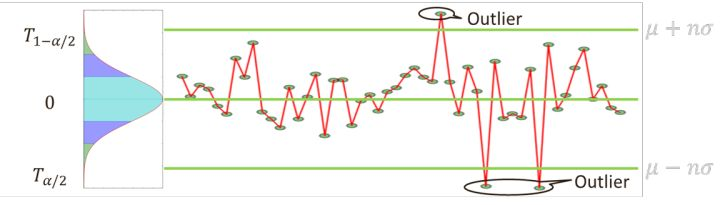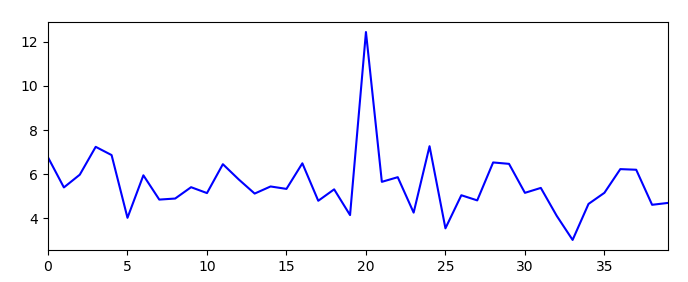# 数据准备
np.random.seed(0)
x = np.arange(40)
y = np.random.normal(loc=5, scale=1, size=len(x))
y += 10
plt.figure(figsize=(8, 3))
pd.Series(data=y, index=x).plot(color='b', linestyle='-')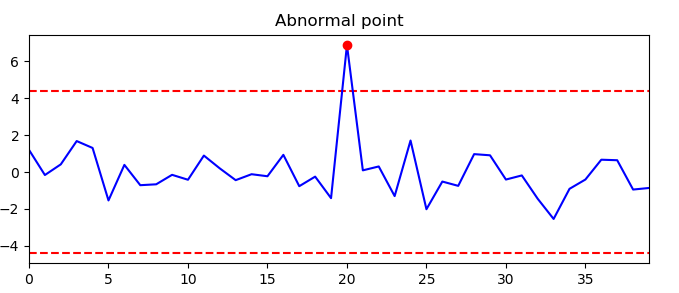# 计算上下线
n = 3
mean = y.mean()
sigma = y.std()
resid = y - mean
up_threshold = n * sigma
low_threshold = -n * sigma
outlier_indexs = [i for i in range(len(resid)) if resid[i] > up_threshold or resid[i] < low_threshold]
outlier_values = resid[outlier_indexs]

plt.title("Abnormal point")
plt.figure(figsize=(8, 3))
pd.Series(data=resid, index=x).plot(color='b', linestyle='-')  # 残差
plt.plot(outlier_indexs, outlier_values, "ro", label="point")  # 异常点
plt.hlines(up_threshold, x, x[-1], colors="r", linestyles="dashed")   # 上线
plt.hlines(low_threshold, x, x[-1], colors="r", linestyles="dashed")  # 下线
plt.show()

#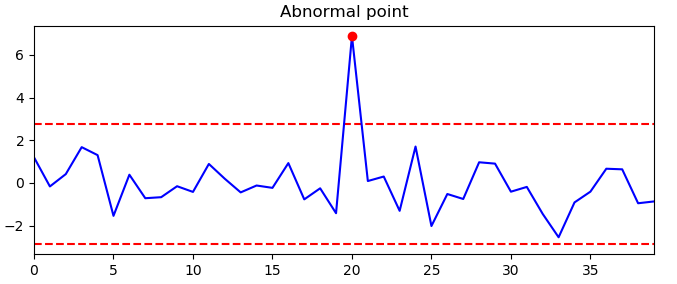# 数据准备
np.random.seed(0)
x = np.arange(40)
y = np.random.normal(loc=5, scale=1, size=len(x))
y += 10
mean = y.mean()
sigma = y.std()
resid = y - mean

Q1 = np.percentile(resid, 25)
Q3 = np.percentile(resid, 75)
IQR = Q3 - Q1
up_threshold = Q3 + 1.5*IQR
low_threshold = Q1 - 1.5*IQR
outlier_indexs = [i for i in range(len(resid)) if resid[i] > up_threshold or resid[i] < low_threshold]
outlier_values = resid[outlier_indexs]

plt.figure(figsize=(8, 3))
plt.title("Abnormal point")
pd.Series(data=resid, index=x).plot(color='b', linestyle='-')  # 残差
plt.plot(outlier_indexs, outlier_values, "ro", label="point")  # 异常点
plt.hlines(up_threshold, x, x[-1], colors="r", linestyles="dashed")   # 上线
plt.hlines(low_threshold, x, x[-1], colors="r", linestyles="dashed")  # 下线
plt.show()

# 5、 异常检测算法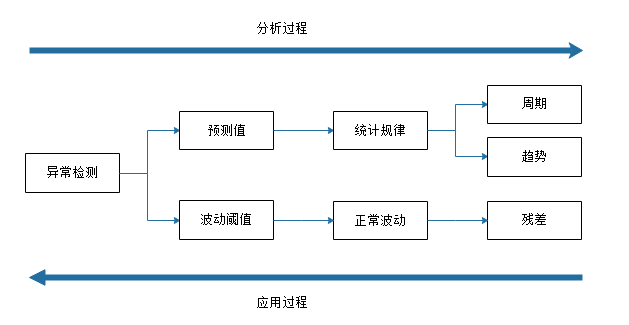序列拟合与预测+异常准则

Step1： 使用 STL 算法分解时序，得到残差；

Step2： 基于 box-plot 准则求得残差的上界和下界；

Step3： 当残差小于Step2中的下界，或者残差大于Step2中的上界，则认为是异常点。

__________________________________

https://www.jianshu.com/p/0aec95162cc6

https://www.cnblogs.com/en-heng/p/7390310.html

https://www.cnblogs.com/runner-ljt/p/5245080.html

https://zhuanlan.zhihu.com/p/142904065

03-132万+

#### 基于时间序列的异常检测算法小结

06-146037

#### 时间序列分解和异常检测方法应用案例09-053万+

#### 大规模时间序列数据自动异常检测架构

10-25498

#### AIOps探索：基于VAE模型的周期性KPI异常检测方法——VAE异常检测

05-15941

#### python中对高维时间序列数据按时间和个体降维成堆积数的方法（求均值）

06-11433

#### 网络KPI异常检测之时序分解算法

07-103421

#### 干货 | 20个教程，掌握时间序列的特征分析（附代码）

06-10100

#### 我觉得一些非常有效的时间序列分析方法，对于KPI异常检测

11-01888

#### KPI自动化异常检测系统——Opprentice

08-123999

#### 关于时间序列中数据的降维简单讨论

07-059370

#### 时间序列聚类方法的研究

07-037111

#### 智能运维（AIOps）系统中的异常检测方案

06-221211

#### 【华为云技术分享】用GaussDB合理管控数据资源的几点心得

06-17232

#### 【华为云-上云之路】网络AI模型开发（3）数据时序标注、数据探索

09-291万+

#### 【时间序列】时间序列分割聚类算法TICC

01-136183

#### 时间序列(time serie)分析系列之时间序列特征(feature)7

06-20449

#### 从618大促聊聊华为云GaussDB NoSQL的蓬勃张力

06-19342

#### 时间序列分析方法——ARIMA模型案例

08-1552万+

#### Python+OpenCV计算机视觉

12-28©️2020 CSDN 皮肤主题: 创作都市 设计师: CSDN官方博客点击重新获取扫码支付1.余额是钱包充值的虚拟货币，按照1:1的比例进行支付金额的抵扣。
2.余额无法直接购买下载，可以购买VIP、C币套餐、付费专栏及课程。余额充值